# 采用有限域克里金方法进行校正条带效应The Correction of String Effect by Finite Domain Kriging

DOI: 10.12677/AG.2018.86115, PDF, HTML, XML, 下载: 606  浏览: 959  国家自然科学基金支持

Abstract: In the process of kriging estimation with finite strings of data, it can be frequently observed that outlying data in the strings receive higher weights than all other data. This counterintuitive weighting is referred as string effect. Taking simple kriging as an example, we adopt the Finite Domain Simple Kriging method (FDSK) to correct the string effect of simple kriging algorithm, and compared with the Distance Constrained Kriging method (DCSK). The FDSK estimators are linear combinations of several traditional kriging estimators. The kriging weights to be used for FDSK are the average of the weights from the traditional kriging runs. FDSK approach has been programmed by FORTRAN language and tested with a small example. The new method can correct the string effect of the traditional kriging method with a high estimate accuracy which has important realistic significance to improve the accuracy of the estimate in the mining and petroleum industries.

1. 引言

2. 简单克里金原理 

${Y}^{*}\left({x}_{0}\right)=\underset{i=1}{\overset{n}{\sum }}{\lambda }_{i}Y\left(xi\right)$

${\sigma }_{E}^{2}=E{\left[Y\left({x}_{0}\right)-{Y}^{*}\left({x}_{0}\right)\right]}^{2}$

$\frac{\partial {\sigma }_{E}^{2}}{\partial {\lambda }_{i}}=-2Cov\left({x}_{i},{x}_{0}\right)+2\underset{j=1}{\overset{n}{\sum }}{\lambda }_{j}Cov\left({x}_{i},{x}_{j}\right)=0$

$\underset{j=1}{\overset{n}{\sum }}{\lambda }_{j}Cov\left({x}_{i},{x}_{j}\right)=Cov\left({x}_{i},{x}_{0}\right)$

${\sigma }_{E}^{2}=Cov\left({x}_{0},{x}_{0}\right)-\underset{i=1}{\overset{n}{\sum }}{\lambda }_{i}Cov\left({x}_{i},{x}_{j}\right)$

3. 简单克里金的改进

3.1. 条带效应

3.2. 有限域克里金方法(FDSK)原理

${Z}_{FDSK}^{*}\left({u}_{0}\right)=\frac{1}{n}\underset{k=1}{\overset{n}{\sum }}\left[{\lambda }_{sk,i}^{k}\left({u}_{0}\right){Z}_{i}^{k}\left({u}_{0}\right)+\left(1-\underset{i=1}{\overset{k}{\sum }}{\lambda }_{sk,i}^{k}\left({u}_{0}\right)\right)m\right]$

$\underset{i=1}{\overset{k}{\sum }}{\lambda }_{sk,i}^{k}Cov\left({Z}_{j}^{k},{Z}_{i}^{k}\right)=Cov\left({Z}_{j}^{k},Z\left({u}_{0}\right)\right),j=1,\cdots ,k$

${Z}_{FDSK}^{*}\left({u}_{0}\right)=\underset{i=1}{\overset{n}{\sum }}{\lambda }_{FDSK,i}\left({u}_{0}\right)+\left(1-\underset{i=1}{\overset{n}{\sum }}{\lambda }_{FDSK,i}\left({u}_{0}\right)\right)m$

${\lambda }_{FDSK,i}=\frac{1}{n}\underset{k=1}{\overset{n}{\sum }}{\stackrel{˜}{\lambda }}_{SK,i}^{k}$

${\sigma }_{FDSK}^{2}\left({u}_{0}\right)={\sigma }^{2}-2\underset{i=1}{\overset{n}{\sum }}{\lambda }_{FDSK,i}Cov\left(Z\left({u}_{i}\right),Z\left({u}_{0}\right)\right)+\underset{i=1}{\overset{n}{\sum }}\underset{j=1}{\overset{n}{\sum }}{\lambda }_{FDSK,i}{\lambda }_{FDSK,j}Cov\left(Z\left({u}_{i}\right),Z\left({u}_{0}\right)\right)$

3.3. FDSK算法实现

FDSK方法实现的具体步骤如下：

1) 计算已知点与待估点之间的一组距离 ${d}_{1},{d}_{2},\cdots ,{d}_{n}$ ，并按升序将这些距离进行排列，令m = (排序后的距离的脚标)；

2) 将已知点按距离进行排列 ${Z}_{1},{Z}_{2},\cdots ,{Z}_{n}$ ；其中，离待估点距离最近的样本点为Z1，离待估点距离次近的样本点为Z2，……，表示离待估点距离最远的样本点为Zn

3) 利用简单克里金方法(SK)求出 $k\left(1,2,\cdots ,n\right)$ 个与待估点最接近的已知点的权值， ${\lambda }_{SK}^{k}=\left({\lambda }_{SK,1}^{k},\cdots ,{\lambda }_{SK,i}^{k}\right),i=1,\cdots ,k$ 并且当 $i>k$ 时，令 ${\lambda }_{SK,i}^{k}=0$

4) 令 ${\lambda }_{F}=\left({\lambda }_{F,1},{\lambda }_{F,2},\cdots ,{\lambda }_{F,n}\right)$

${\lambda }_{F,i}=\frac{1}{n}\underset{k=1}{\overset{n}{\sum }}{\lambda }_{SK,m\left(i\right)}^{k}$

5) 则 ${\lambda }_{F}$ 中的元就是我们所需要的FDSK权值。

4. 权值计算检验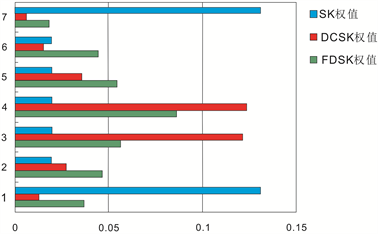Figure 1. Weights with a nugget effect of 0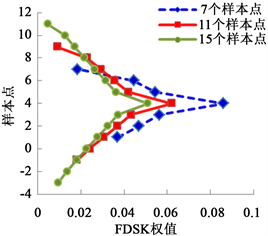(a)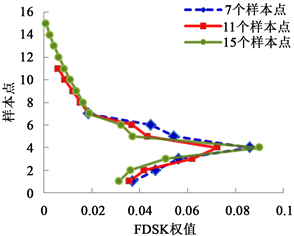(b)

Figure 2. Weights with different numbers of Strings of data. (a) The number of conditional data increases to both ends; (b) The number of conditional data increases toward one end

5. 数值模拟计算检验(a)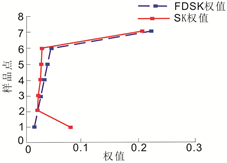(b)

Figure 3. Weights in different locations of the estimation point. (a) An estimate of the point to be estimated at (1, 20); (b) An estimate of the point to be estimated at (7, 20)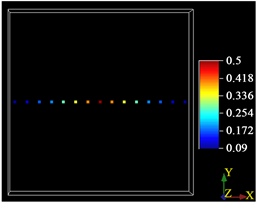Figure 4. Strings of data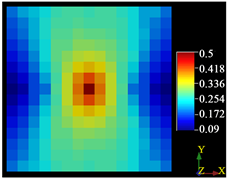(a)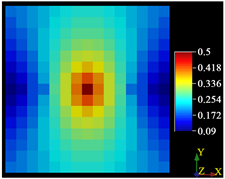(b)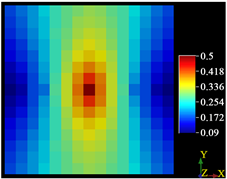(c)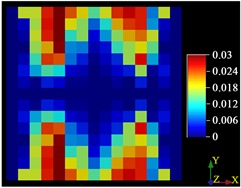(d)(e)

Figure 5. Simulation results. (a) Results of DCSK simulation; (b) Results of SK simulation; (c) Results of FDSK simulation; (d) Difference map of simulation results between DCSK and SK; (e) Difference map of simulation results between FDSK and SK

6. 实测数据应用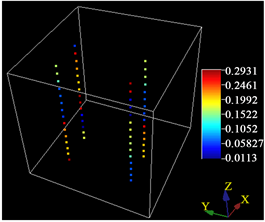Figure 6. Porosity data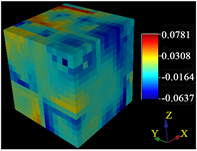(a)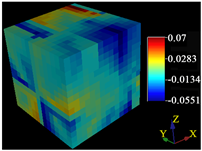(b)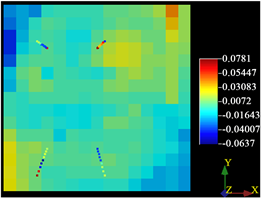(c)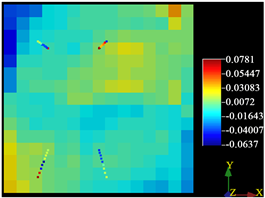(d)

Figure 7. Simulation results. (a) Difference Diagram of Simulation Results between DCSK and SK; (b) Difference map of simulation results between FDSK and SK; (c) Differential Slice Map of DCSK in Z Direction; (d) Differential slice of FDSK in Z direction

7. 结论

NOTES

*通讯作者。

  Deutsch, C.V. and Journel, A.G. (1998) GSLIB: Geostatistical Software Library: And User’s Guide. 2nd Edition, Oxford University Press, New York.  Deutsch, C.V. (2002) Geostatistical Reservoir Modeling. Oxford University Press, New York.  Deutsch, C.V. (1994) Kriging with Strings of Data. Mathematical Geology, 26, 623-638. https://doi.org/10.1007/BF02089245  Babak, O. (2008) Theoretical and Practical Improvements to Geostatistical Estimation and Simulation. University of Alberta, Edmonton.  郑光辉, 黄克龙, 张志宏, 等. 运用克里金空间插值技术进行土地级别划分[J]. 南京师大学报(自然科学版), 2007, 30(1): 112-116.  杜国明, 汪光松, 吴超羽, 等. 克里金在珠江河道地形空间数据内插中的应用[J]. 中山大学学报(自然科学版), 2007, 46(1): 119-122.  杨功流, 张桂敏, 李士心. 泛克里金插值法在地磁图中的应用[J]. 中国惯性技术学报, 2008, 16(2): 162-166.  陈亮, 黄述旺, 赖泽武. 应用截断高斯随机模拟法研究沉积微相[J]. 石油勘探与开发, 1998, 25(6): 78-80.  纪发华, 熊琦华. 序贯指示建模方法在枣南油田储层非均质研究中的应用[J]. 石油学报, 1994, 15(增刊): 179-186.  徐景帧, 刘晓冬, 等. 渗透率建模的三维指示条件模拟方法及其应用[J]. 石油学报, 2000, 21(3): 61-65.  李少华, 张昌民, 尹艳树. 储层建模算法剖析[M]. 北京: 石油工业出版社, 2012: 36-37.